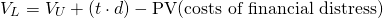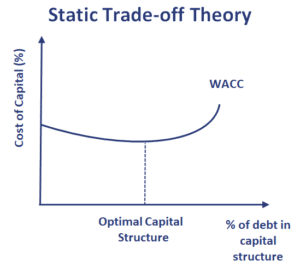The static trade-off theory of the capital structure is a theory of the capital structure of firms. The theory tries to balance the costs of financial distress with the tax shield benefit from using debt. Under this theory, there exists an optimal capital structure that is a combination of debt and equity. In fact, the trade-off theory relaxes a number of assumptions under the capital structure irrelevance theory of Merton Miller and Franco Modigliani.

On this page, we discuss the static trade-off theory and how the optimal level of debt can be determined. We also visualize the relationship with the Weighted Average Cost of Capital (WACC).

The trade-off theory starts from the capital structure irrelevance theory, but relaxes one of the assumptions. The theory removes the assumption that there are no costs to financial distress when the companies borrows more money. If we remove that assumption, then taking on more debt does not necessarily lower the WACC. Instead, it there will be a point at which the additional value added from taking on more debt (tax shield) is exceeded by the value-reducing cost of financial distress.

This point is the optimal capital structure and it is the point where the value of the firm is maximized.

The static trade-off theory formula looks as followswhere VL is the value of the leveraged firm, VU is the value of the unlevered firm, t is the marginal tax rate, and d is the value of the debt.

In the following figure, we show the relationship between the cost of capital and the % of debt in the capital structure. We stee that the after-tax cost of debt as an upward slope because of the increasing cost of financial distress. As the cost of the debt increases, so does the cost of equity. The optimal point is where the marginal benefit of the tax shield equals the marginal cost of financial distressAn alternative method that is sometimes used to illustrate the relationship is by plotting the firm value and the % of debt in the capital structure. In that case, there will be a point on the curve where the value of the levered firms is highest. This is the point where the capital structure is optimal.

## Summary

We discussed the static trade-off theory, a theory of the capital structure that is better able to explain companies’ capital structure in practice than Miller and Modigliani’s capital irrelevance propositions.